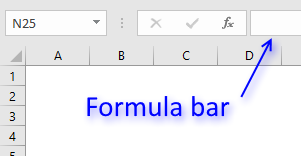Author: Oscar Cronquist Article last updated on February 01, 2019

### Question:

How to calculate the date of the third Monday of a given month?

Column B contains dates of the first date of a month, however, they are formatted as Month and year. This makes it possible to use the corresponding cell value in our formula.

Array formula in cell C3

=SMALL(IF(WEEKDAY(B3 +ROW(INDIRECT( "\$1:\$"&DAY( EOMONTH(B3, 0))))-1)=2, B3+ROW( INDIRECT("\$1:\$"&DAY( EOMONTH(B3, 0))))-1, ""), 3)

To enter an array formula, type the formula in cell C3 then press and hold CTRL + SHIFT simultaneously, now press Enter once. Release all keys.The formula bar now shows the formula with a beginning and ending curly bracket telling you that you entered the formula successfully.

Don't enter the curly brackets yourself, they appear automatically.

### Explaining array formula in cell C3

#### Step 1 - Create an array of numbers corresponding to days in month

The EOMONTH function calculates the last date in a given month, the DAY function then returns the day of that date.

ROW(INDIRECT( "\$1:\$"&DAY( EOMONTH(B3, 0))))-1)

becomes

ROW(INDIRECT( "\$1:\$"&DAY( EOMONTH(39814, 0))))-1)

becomes

ROW(INDIRECT( "\$1:\$"&DAY( 39844)))-1)

becomes

ROW(INDIRECT( "\$1:\$"&DAY( 39844)))-1)

becomes

ROW(INDIRECT("\$1:\$"&31))-1

The INDIRECT function converts a text string to a cell reference that an Excel function then can use.

ROW(INDIRECT(\$1:\$31))-1

becomes

ROW(\$1:\$31)-1

The ROW function then returns the row numbers of each cell in the cell reference.

ROW(\$1:\$31)-1

becomes

{1; 2; 3; 4; 5; 6; 7; 8; 9; 10; 11; 12; 13; 14; 15; 16; 17; 18; 19; 20; 21; 22; 23; 24; 25; 26; 27; 28; 29; 30; 31} - 1

and returns {0; 1; 2; 3; 4; 5; 6; 7; 8; 9; 10; 11; 12; 13; 14; 15; 16; 17; 18; 19; 20; 21; 22; 23; 24; 25; 26; 27; 28; 29; 30}

#### Step 2 - Check if date is a Monday

The array in the step before is now going to be added to the first date in the given month.

WEEKDAY(B3 +ROW(INDIRECT( "\$1:\$"&DAY( EOMONTH(B3, 0))))-1)=2

becomes

WEEKDAY(B3 +{0; 1; 2; 3; 4; 5; 6; 7; 8; 9; 10; 11; 12; 13; 14; 15; 16; 17; 18; 19; 20; 21; 22; 23; 24; 25; 26; 27; 28; 29; 30})=2

becomes

WEEKDAY({39814; 39815; 39816; 39817; 39818; 39819; 39820; 39821; 39822; 39823; 39824; 39825; 39826; 39827; 39828; 39829; 39830; 39831; 39832; 39833; 39834; 39835; 39836; 39837; 39838; 39839; 39840; 39841; 39842; 39843; 39844})=2

It is worth mentioning that Excel treats dates as numbers. For example, number 1 is 1/1/1900 and 1/1/2000 is 36526. 1/2/2000 is 36527. The numbers you see above in the array are dates.

The WEEKDAY function converts the dates to their corresponding weekdays in numbers. 1 is Sunday and 7 is Saturday.

WEEKDAY({39814; 39815; 39816; 39817; 39818; 39819; 39820; 39821; 39822; 39823; 39824; 39825; 39826; 39827; 39828; 39829; 39830; 39831; 39832; 39833; 39834; 39835; 39836; 39837; 39838; 39839; 39840; 39841; 39842; 39843; 39844})=2

becomes

{5;6;7;1;2;3;4;5;6;7;1;2;3;4;5;6;7;1;2;3;4;5;6;7;1;2;3;4;5;6;7}=2

To identify Mondays in this array I will compare the numbers with 2.

{5;6;7;1;2;3;4;5;6;7;1;2;3;4;5;6;7;1;2;3;4;5;6;7;1;2;3;4;5;6;7}=2

returns the following boolean array:

{FALSE; FALSE; FALSE; FALSE; TRUE; FALSE; FALSE; FALSE; FALSE; FALSE; FALSE; TRUE; FALSE; FALSE; FALSE; FALSE; FALSE; FALSE; TRUE; FALSE; FALSE; FALSE; FALSE; FALSE; FALSE; TRUE; FALSE; FALSE; FALSE; FALSE; FALSE}

Boolean values are TRUE and FALSE.

Step 3 - Return corresponding date if Monday

The IF function lets you evaluate a logical expression and if TRUE one thing happens and if FALSE another thing happens.

The logical expression in this example evaluates to TRUE if date is a Monday.

IF(WEEKDAY(B3+ROW(INDIRECT("\$1:\$"&DAY(EOMONTH(B3,0))))-1)=2,B3+ROW(INDIRECT("\$1:\$"&DAY(EOMONTH(B3,0))))-1,"")

becomes

IF({FALSE; FALSE; FALSE; FALSE; TRUE; FALSE; FALSE; FALSE; FALSE; FALSE; FALSE; TRUE; FALSE; FALSE; FALSE; FALSE; FALSE; FALSE; TRUE; FALSE; FALSE; FALSE; FALSE; FALSE; FALSE; TRUE; FALSE; FALSE; FALSE; FALSE; FALSE},B3+ROW(INDIRECT("\$1:\$"&DAY(EOMONTH(B3,0))))-1,"")

If the value is TRUE then return the corresponding date. Since months have different number of days we must build an array that takes that into account.

It works just like the array we created in step 1.

IF({FALSE; FALSE; FALSE; FALSE; TRUE; FALSE; FALSE; FALSE; FALSE; FALSE; FALSE; TRUE; FALSE; FALSE; FALSE; FALSE; FALSE; FALSE; TRUE; FALSE; FALSE; FALSE; FALSE; FALSE; FALSE; TRUE; FALSE; FALSE; FALSE; FALSE; FALSE},B3+ROW(INDIRECT("\$1:\$"&DAY(EOMONTH(B3,0))))-1,"")

becomes

IF({FALSE; FALSE; FALSE; FALSE; TRUE; FALSE; FALSE; FALSE; FALSE; FALSE; FALSE; TRUE; FALSE; FALSE; FALSE; FALSE; FALSE; FALSE; TRUE; FALSE; FALSE; FALSE; FALSE; FALSE; FALSE; TRUE; FALSE; FALSE; FALSE; FALSE; FALSE},{39814; 39815; 39816; 39817; 39818; 39819; 39820; 39821; 39822; 39823; 39824; 39825; 39826; 39827; 39828; 39829; 39830; 39831; 39832; 39833; 39834; 39835; 39836; 39837; 39838; 39839; 39840; 39841; 39842; 39843; 39844},"")

and returns

{""; ""; ""; ""; 39818; ""; ""; ""; ""; ""; ""; 39825; ""; ""; ""; ""; ""; ""; 39832; ""; ""; ""; ""; ""; ""; 39839; ""; ""; ""; ""; ""}

Step 3 - Filter third Monday

The SMALL function lets you return the third largest number in array because we are looking for the third Monday in a given month.

SMALL(IF(WEEKDAY(B3 +ROW(INDIRECT( "\$1:\$"&DAY( EOMONTH(B3, 0))))-1)=2, B3+ROW( INDIRECT("\$1:\$"&DAY( EOMONTH(B3, 0))))-1, ""), 3)

becomes

SMALL({""; ""; ""; ""; 39818; ""; ""; ""; ""; ""; ""; 39825; ""; ""; ""; ""; ""; ""; 39832; ""; ""; ""; ""; ""; ""; 39839; ""; ""; ""; ""; ""}, 3)

and returns 39832 in cell C3 which is the same as 1/19/2009 if formatted as a date in Excel.